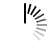Private Destructor in C++

Here we will see what will be the case if the destructors are private in C++. Let us see some example codes to get the idea.

This code has private destructor, but it will not generate any error because no object is created.

Example

```#include <iostream>
using namespace std;
class my_class {
private:
~my_class(){
//private destructor
}
};
int main() {
}```

In this program, this will generate compilation error, as we are trying to create one object, but the compiler can notice that the destructor is not accessible. So it cannot be destroyed after completing the task.

Example

```#include <iostream>
using namespace std;
class my_class {
private:
~my_class() {
//private destructor
}
};
int main() {
my_class obj;
}```

Output

`[Error] 'my_class::~my_class()' is private`

Now if we create one pointer for that class, then it will not generate error because no actual object is created.

Example

```#include <iostream>
using namespace std;
class my_class {
private:
~my_class() {
//private destructor
}
};
int main() {
my_class *obj;
}```

If one object is created using the new operator, then also no error will generate. The compiler thinks that this is programmer’s responsibility to delete the object from memory.

Example

```#include <iostream>
using namespace std;
class my_class {
private:
~my_class() {
//private destructor
}
};
int main() {
my_class *obj = new my_class;
}```

Output

Now if we add delete statement to delete the object, it will generate error for the private destructor.

Example

```#include <iostream>
using namespace std;
class my_class {
private:
~my_class() {
//private destructor
}
};
int main() {
my_class *obj = new my_class;
delete obj;
}```

Output

`[Error] 'my_class::~my_class()' is private`